# How to Use the TRIM Function in ExcelClaudia Buckley#### Join the Excel conversation on Slack

Ask a question or join the conversation for all things Excel on our Slack channel.

Excel is only as valuable as the data that is entered into it. Data that is irregular or inconsistent can give inaccurate results. An innocent-looking space character, for example, can cause a worksheet to tell an entirely different story.

## What does the TRIM function do?

The purpose of the TRIM function in Excel is usually to clean up data as a first step to getting it ready for analysis. TRIM removes spaces from text except for single spaces between words. When text gets imported from another application, spacing is often irregular and may include leading, trailing, or extra spaces between words. At other times, incorrect spacing may result from input errors.

## TRIM function syntax

The format of the TRIM function is quite simple. It is:

``=TRIM(text)``

The only argument is the text to be trimmed, and Excel takes care of the rest. Text may refer to a text string entered within double quotes or to a cell reference containing a text string.

## Basic application

A simple example of how TRIM works is shown below.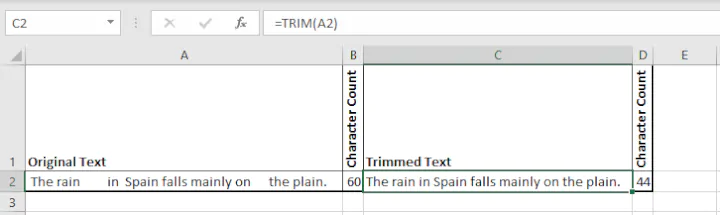The original text (in cell A2) contained 60 characters because of all the extra space characters. After using the TRIM function, the return value in cell C2 shows the number of characters reduced to 44. The extra spaces in the middle of the sentence were pretty obvious, but TRIM also removed leading spaces and trailing spaces that might have been missed.

Note that the TRIM function is only meant to work with the ASCII space character (32). It does not affect the non-breaking space special character.

Use this free TRIM Excel file to practice along with the tutorial.

## Examples - using TRIM to solve problems

The TRIM function is seldom used on its own. More often, it is used to support other text functions so that they return accurate results. A few examples are below.

### Split data with the help of the TRIM function

For example, the LEFT, RIGHT, and MID functions often extract specific data fragments from a text string. In the example below, we might use the RIGHT function to get the postal codes from each address. We may know that postal codes are seven characters long, including the space in the middle, so we expect that the format

``=RIGHT(text,7)``

will get us the desired result.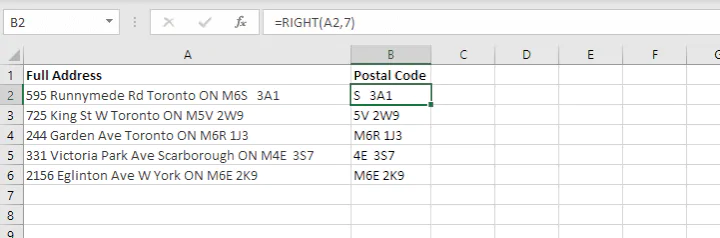However, it does not because there is uneven spacing in several rows. To fix this, we can trim the text before using RIGHT to do the extraction. Nesting (embedding) one function within another allows us to do this in a single formula.

It could be reasoned that we need to trim the text string before extracting the last seven characters, so we will embed the TRIM function within the RIGHT function so that Excel processes that result first. Our formula is as follows:

````=RIGHT(TRIM(A2),7)`
```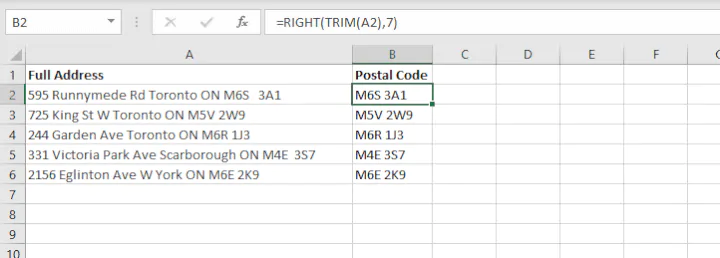The same principle applies to the LEFT, MID, or LEN function. The TRIM function can be used as the text argument to get the desired result, after which other functions can be applied. Nest functions allow us to do both steps in one entry.

### Concatenate values with the help of the TRIM function

We might face a similar problem when trying to reverse - join values across several cells into a single cell. In the example below, the CONCAT function joins first and last names from columns A and B. A space character should separate each name, but the values returned show that the original text had several instances of leading spaces and even trailing spaces, which are harder to catch.

````=CONCAT(A2, “ ”,B2)`
```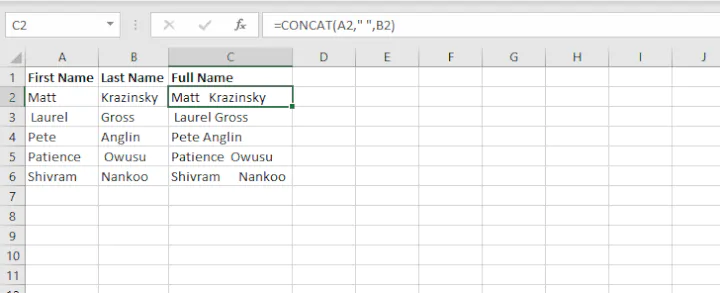In this case, we should perform the concatenation operation first, then trim the result. So CONCAT becomes the inner function.

``=TRIM(CONCAT(A2,“ ”,B2))``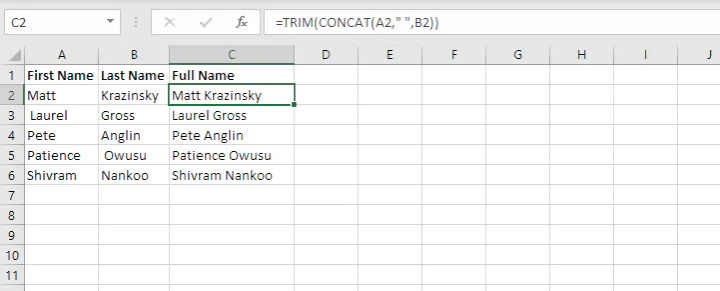After the values are joined, we trim the result to ensure that there are no extra space characters.

### Use TRIM to determine the number of words in a string

The LEN and SUBSTITUTE functions may be used together to determine the number of words in a cell. Since space characters separate words, this formula compares the length of a text string before and after the removal of space characters.

The standard formula is

````=LEN(text)-LEN(SUBSTITUTE(text," ",""))+1`
```

The graphic below describes the logic of the formula.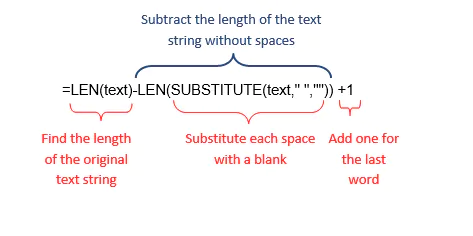You can see, however, the problem that would result if the original text had irregular spacing. Excel would assume that each space character represented a word, which would return a higher number of words than is the case.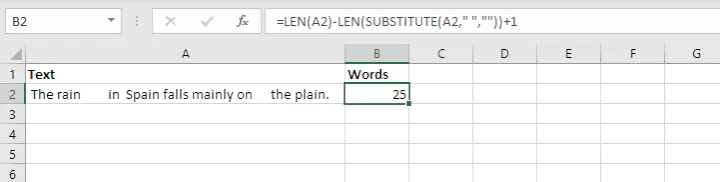Again, TRIM comes to the rescue. We quickly solve that problem by trimming the original text before finding its length.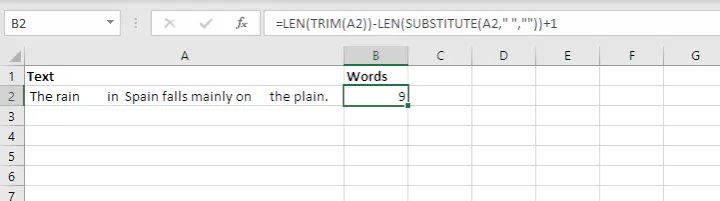So simple, but so effective!

### Using TRIM with numeric values

TRIM is considered a text function. Though it can also be used to trim numeric values, these values may not behave as expected after TRIM has been applied.

In the example below, the original string has been trimmed, and then the MID function is used to eliminate the first seven characters, which consist of the 5-character item code, followed by a space, a dash, and another space.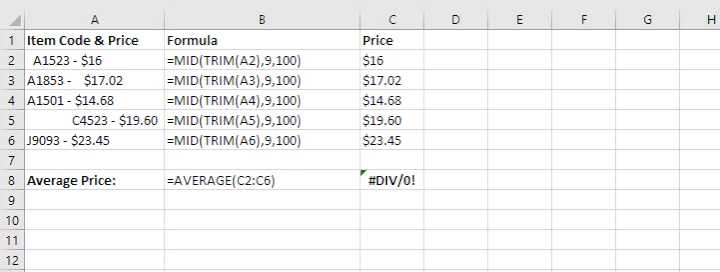The values in the range C2:C6 appear as numbers but are actually stored as text. One clue is that they are aligned to the left, the default alignment for text values. This results in a #DIV/0! error response when we try to find the average of these numbers. (See the response from the AVERAGE formula entered in cell C8.) Since text values are considered non-numeric, Excel determines that we are attempting to divide by zero.

The way to fix this is to convert these values into numbers with the VALUE function. The cells can then be formatted as currency, and the average price can be calculated as expected.

``=VALUE(MID(TRIM(A2),9,100))``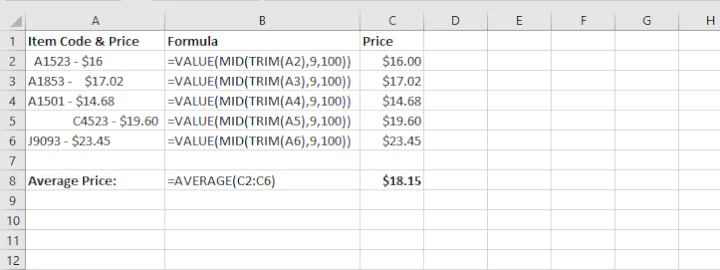## Final word

Now that you know how to use the TRIM function, you can see why many people consider it one of the need-to-know functions for every Excel user. Check out our resource library to learn more.

Ready to become a certified Excel ninja?